Professor Murray

Word Problems and Applications of Trigonometry

Slide Duration:

Section 1: Trigonometric Functions
Angles

39m 5s

Intro
0:00
Degrees
0:22
Circle is 360 Degrees
0:48
Splitting a Circle
1:13
2:08
2:31
2:52
Half-Circle and Right Angle
4:00
6:24
6:52
Coterminal, Complementary, Supplementary Angles
7:23
Coterminal Angles
7:30
Complementary Angles
9:40
Supplementary Angles
10:08
Example 1: Dividing a Circle
10:38
Example 2: Converting Between Degrees and Radians
11:56
Example 3: Quadrants and Coterminal Angles
14:18
Extra Example 1: Common Angle Conversions
-1
Extra Example 2: Quadrants and Coterminal Angles
-2
Sine and Cosine Functions

43m 16s

Intro
0:00
Sine and Cosine
0:15
Unit Circle
0:22
Coordinates on Unit Circle
1:03
Right Triangles
1:52
2:25
Master Right Triangle Formula: SOHCAHTOA
2:48
Odd Functions, Even Functions
4:40
Example: Odd Function
4:56
Example: Even Function
7:30
Example 1: Sine and Cosine
10:27
Example 2: Graphing Sine and Cosine Functions
14:39
Example 3: Right Triangle
21:40
Example 4: Odd, Even, or Neither
26:01
Extra Example 1: Right Triangle
-1
Extra Example 2: Graphing Sine and Cosine Functions
-2
Sine and Cosine Values of Special Angles

33m 5s

Intro
0:00
45-45-90 Triangle and 30-60-90 Triangle
0:08
45-45-90 Triangle
0:21
30-60-90 Triangle
2:06
Mnemonic: All Students Take Calculus (ASTC)
5:21
Using the Unit Circle
5:59
New Angles
6:21
9:43
Mnemonic: All Students Take Calculus
10:13
13:11
16:48
Example 3: All Angles and Quadrants
20:21
Extra Example 1: Convert, Quadrant, Sine/Cosine
-1
Extra Example 2: All Angles and Quadrants
-2
Modified Sine Waves: Asin(Bx+C)+D and Acos(Bx+C)+D

52m 3s

Intro
0:00
Amplitude and Period of a Sine Wave
0:38
Sine Wave Graph
0:58
Amplitude: Distance from Middle to Peak
1:18
Peak: Distance from Peak to Peak
2:41
Phase Shift and Vertical Shift
4:13
Phase Shift: Distance Shifted Horizontally
4:16
Vertical Shift: Distance Shifted Vertically
6:48
Example 1: Amplitude/Period/Phase and Vertical Shift
8:04
Example 2: Amplitude/Period/Phase and Vertical Shift
17:39
Example 3: Find Sine Wave Given Attributes
25:23
Extra Example 1: Amplitude/Period/Phase and Vertical Shift
-1
Extra Example 2: Find Cosine Wave Given Attributes
-2
Tangent and Cotangent Functions

36m 4s

Intro
0:00
Tangent and Cotangent Definitions
0:21
Tangent Definition
0:25
Cotangent Definition
0:47
Master Formula: SOHCAHTOA
1:01
Mnemonic
1:16
Tangent and Cotangent Values
2:29
Remember Common Values of Sine and Cosine
2:46
90 Degrees Undefined
4:36
Slope and Menmonic: ASTC
5:47
Uses of Tangent
5:54
Example: Tangent of Angle is Slope
6:09
7:49
Example 1: Graph Tangent and Cotangent Functions
10:42
Example 2: Tangent and Cotangent of Angles
16:09
Example 3: Odd, Even, or Neither
18:56
Extra Example 1: Tangent and Cotangent of Angles
-1
Extra Example 2: Tangent and Cotangent of Angles
-2
Secant and Cosecant Functions

27m 18s

Intro
0:00
Secant and Cosecant Definitions
0:17
Secant Definition
0:18
Cosecant Definition
0:33
Example 1: Graph Secant Function
0:48
Example 2: Values of Secant and Cosecant
6:49
Example 3: Odd, Even, or Neither
12:49
Extra Example 1: Graph of Cosecant Function
-1
Extra Example 2: Values of Secant and Cosecant
-2
Inverse Trigonometric Functions

32m 58s

Intro
0:00
Arcsine Function
0:24
Restrictions between -1 and 1
0:43
Arcsine Notation
1:26
Arccosine Function
3:07
Restrictions between -1 and 1
3:36
Cosine Notation
3:53
Arctangent Function
4:30
Between -Pi/2 and Pi/2
4:44
Tangent Notation
5:02
Example 1: Domain/Range/Graph of Arcsine
5:45
Example 2: Arcsin/Arccos/Arctan Values
10:46
Example 3: Domain/Range/Graph of Arctangent
17:14
Extra Example 1: Domain/Range/Graph of Arccosine
-1
Extra Example 2: Arcsin/Arccos/Arctan Values
-2
Computations of Inverse Trigonometric Functions

31m 8s

Intro
0:00
Inverse Trigonometric Function Domains and Ranges
0:31
Arcsine
0:41
Arccosine
1:14
Arctangent
1:41
Example 1: Arcsines of Common Values
2:44
Example 2: Odd, Even, or Neither
5:57
Example 3: Arccosines of Common Values
12:24
Extra Example 1: Arctangents of Common Values
-1
Extra Example 2: Arcsin/Arccos/Arctan Values
-2
Section 2: Trigonometric Identities
Pythagorean Identity

19m 11s

Intro
0:00
Pythagorean Identity
0:17
Pythagorean Triangle
0:27
Pythagorean Identity
0:45
Example 1: Use Pythagorean Theorem to Prove Pythagorean Identity
1:14
Example 2: Find Angle Given Cosine and Quadrant
4:18
Example 3: Verify Trigonometric Identity
8:00
Extra Example 1: Use Pythagorean Identity to Prove Pythagorean Theorem
-1
Extra Example 2: Find Angle Given Cosine and Quadrant
-2
Identity Tan(squared)x+1=Sec(squared)x

23m 16s

Intro
0:00
Main Formulas
0:19
Companion to Pythagorean Identity
0:27
For Cotangents and Cosecants
0:52
How to Remember
0:58
Example 1: Prove the Identity
1:40
Example 2: Given Tan Find Sec
3:42
Example 3: Prove the Identity
7:45
Extra Example 1: Prove the Identity
-1
Extra Example 2: Given Sec Find Tan
-2

52m 52s

Intro
0:00
0:09
How to Remember
0:48
Cofunction Identities
1:31
How to Remember Graphically
1:44
Where to Use Cofunction Identities
2:52
Example 1: Derive the Formula for cos(A-B)
3:08
Example 2: Use Addition and Subtraction Formulas
16:03
Example 3: Use Addition and Subtraction Formulas to Prove Identity
25:11
Extra Example 1: Use cos(A-B) and Cofunction Identities
-1
Extra Example 2: Convert to Radians and use Formulas
-2
Double Angle Formulas

29m 5s

Intro
0:00
Main Formula
0:07
How to Remember from Addition Formula
0:18
Two Other Forms
1:35
Example 1: Find Sine and Cosine of Angle using Double Angle
3:16
Example 2: Prove Trigonometric Identity using Double Angle
9:37
Example 3: Use Addition and Subtraction Formulas
12:38
Extra Example 1: Find Sine and Cosine of Angle using Double Angle
-1
Extra Example 2: Prove Trigonometric Identity using Double Angle
-2
Half-Angle Formulas

43m 55s

Intro
0:00
Main Formulas
0:09
Confusing Part
0:34
Example 1: Find Sine and Cosine of Angle using Half-Angle
0:54
Example 2: Prove Trigonometric Identity using Half-Angle
11:51
Example 3: Prove the Half-Angle Formula for Tangents
18:39
Extra Example 1: Find Sine and Cosine of Angle using Half-Angle
-1
Extra Example 2: Prove Trigonometric Identity using Half-Angle
-2
Section 3: Applications of Trigonometry
Trigonometry in Right Angles

25m 43s

Intro
0:00
Master Formula for Right Angles
0:11
SOHCAHTOA
0:15
Only for Right Triangles
1:26
Example 1: Find All Angles in a Triangle
2:19
Example 2: Find Lengths of All Sides of Triangle
7:39
Example 3: Find All Angles in a Triangle
11:00
Extra Example 1: Find All Angles in a Triangle
-1
Extra Example 2: Find Lengths of All Sides of Triangle
-2
Law of Sines

56m 40s

Intro
0:00
Law of Sines Formula
0:18
SOHCAHTOA
0:27
Any Triangle
0:59
Graphical Representation
1:25
Solving Triangle Completely
2:37
When to Use Law of Sines
2:55
ASA, SAA, SSA, AAA
2:59
SAS, SSS for Law of Cosines
7:11
Example 1: How Many Triangles Satisfy Conditions, Solve Completely
8:44
Example 2: How Many Triangles Satisfy Conditions, Solve Completely
15:30
Example 3: How Many Triangles Satisfy Conditions, Solve Completely
28:32
Extra Example 1: How Many Triangles Satisfy Conditions, Solve Completely
-1
Extra Example 2: How Many Triangles Satisfy Conditions, Solve Completely
-2
Law of Cosines

49m 5s

Intro
0:00
Law of Cosines Formula
0:23
Graphical Representation
0:34
Relates Sides to Angles
1:00
Any Triangle
1:20
Generalization of Pythagorean Theorem
1:32
When to Use Law of Cosines
2:26
SAS, SSS
2:30
Heron's Formula
4:49
Semiperimeter S
5:11
Example 1: How Many Triangles Satisfy Conditions, Solve Completely
5:53
Example 2: How Many Triangles Satisfy Conditions, Solve Completely
15:19
Example 3: Find Area of a Triangle Given All Side Lengths
26:33
Extra Example 1: How Many Triangles Satisfy Conditions, Solve Completely
-1
Extra Example 2: Length of Third Side and Area of Triangle
-2
Finding the Area of a Triangle

27m 37s

Intro
0:00
Master Right Triangle Formula and Law of Cosines
0:19
SOHCAHTOA
0:27
Law of Cosines
1:23
Heron's Formula
2:22
Semiperimeter S
2:37
Example 1: Area of Triangle with Two Sides and One Angle
3:12
Example 2: Area of Triangle with Three Sides
6:11
Example 3: Area of Triangle with Three Sides, No Heron's Formula
8:50
Extra Example 1: Area of Triangle with Two Sides and One Angle
-1
Extra Example 2: Area of Triangle with Two Sides and One Angle
-2
Word Problems and Applications of Trigonometry

34m 25s

Intro
0:00
Formulas to Remember
0:11
SOHCAHTOA
0:15
Law of Sines
0:55
Law of Cosines
1:48
Heron's Formula
2:46
Example 1: Telephone Pole Height
4:01
Example 2: Bridge Length
7:48
Example 3: Area of Triangular Field
14:20
Extra Example 1: Kite Height
-1
Extra Example 2: Roads to a Town
-2
Vectors

46m 42s

Intro
0:00
Vector Formulas and Concepts
0:12
Vectors as Arrows
0:28
Magnitude
0:38
Direction
0:50
Drawing Vectors
1:16
Uses of Vectors: Velocity, Force
1:37
Vector Magnitude Formula
3:15
Vector Direction Formula
3:28
Vector Components
6:27
Example 1: Magnitude and Direction of Vector
8:00
Example 2: Force to a Box on a Ramp
12:25
Example 3: Plane with Wind
18:30
Extra Example 1: Components of a Vector
-1
Extra Example 2: Ship with a Current
-2
Section 4: Complex Numbers and Polar Coordinates
Polar Coordinates

1h 7m 35s

Intro
0:00
Polar Coordinates vs Rectangular/Cartesian Coordinates
0:12
Rectangular Coordinates, Cartesian Coordinates
0:23
Polar Coordinates
0:59
Converting Between Polar and Rectangular Coordinates
2:06
R
2:16
Theta
2:48
Example 1: Convert Rectangular to Polar Coordinates
6:53
Example 2: Convert Polar to Rectangular Coordinates
17:28
Example 3: Graph the Polar Equation
28:00
Extra Example 1: Convert Polar to Rectangular Coordinates
-1
Extra Example 2: Graph the Polar Equation
-2
Complex Numbers

35m 59s

Intro
0:00
Main Definition
0:07
Number i
0:23
Complex Number Form
0:33
Powers of Imaginary Number i
1:00
Repeating Pattern
1:43
Operations on Complex Numbers
3:30
3:39
Multiplying Complex Numbers
4:39
FOIL Method
5:06
Conjugation
6:29
Dividing Complex Numbers
7:34
Conjugate of Denominator
7:45
Example 1: Solve For Complex Number z
11:02
Example 2: Expand and Simplify
15:34
Example 3: Simplify the Powers of i
17:50
Extra Example 1: Simplify
-1
Extra Example 2: All Complex Numbers Satisfying Equation
-2
Polar Form of Complex Numbers

40m 43s

Intro
0:00
Polar Coordinates
0:49
Rectangular Form
0:52
Polar Form
1:25
R and Theta
1:51
Polar Form Conversion
2:27
R and Theta
2:35
Optimal Values
4:05
Euler's Formula
4:25
Multiplying Two Complex Numbers in Polar Form
6:10
Multiply r's Together and Add Exponents
6:32
Example 1: Convert Rectangular to Polar Form
7:17
Example 2: Convert Polar to Rectangular Form
13:49
Example 3: Multiply Two Complex Numbers
17:28
Extra Example 1: Convert Between Rectangular and Polar Forms
-1
Extra Example 2: Simplify Expression to Polar Form
-2
DeMoivre's Theorem

57m 37s

Intro
0:00
Introduction to DeMoivre's Theorem
0:10
n nth Roots
3:06
DeMoivre's Theorem: Finding nth Roots
3:52
Relation to Unit Circle
6:29
One nth Root for Each Value of k
7:11
Example 1: Convert to Polar Form and Use DeMoivre's Theorem
8:24
Example 2: Find Complex Eighth Roots
15:27
Example 3: Find Complex Roots
27:49
Extra Example 1: Convert to Polar Form and Use DeMoivre's Theorem
-1
Extra Example 2: Find Complex Fourth Roots
-2
Bookmark & Share Embed

## Copy & Paste this embed code into your website’s HTML

Please ensure that your website editor is in text mode when you paste the code.
(In Wordpress, the mode button is on the top right corner.)
×
• - Allow users to view the embedded video in full-size.
Since this lesson is not free, only the preview will appear on your website.

• ## Related Books1 answerLast reply by: Dr. William MurrayTue Jan 7, 2014 11:55 AMPost by Anwar Alasmari on January 1, 2014Hello Doctor,in the example III, why the result of the root of 350(190)(110)(50) is equals 100 times root of 35(19)(11)(5)?3 answersLast reply by: Dr. William MurrayTue Aug 13, 2013 5:17 PMPost by Taylor Wright on July 19, 2013I thought that the Law of Sines doesn't work for AAA, because there would be infinite solutions.

### Word Problems and Applications of Trigonometry

Main formulas:

• Master formula for right triangles: SOHCAHTOA!
• The Law of Sines (in any triangle)
• The Law of Cosines (in any triangle)
• Heron's Formula (in any triangle)

Example 1:

A telephone pole casts a shadow 20 feet long. If the sun's rays make a 60°  angle with the ground, how tall is the pole?

Example 2:

Civil engineers are planning to build a bridge across a lake, but they can't measure the width of the lake directly. They measure from a point on land that is 280 feet from one end of the planned bridge and 160 feet from the other. If the angle between these two lines measures 80° , then how long will the bridge be?

Example 3:

A farmer measures the fences along the edges of a triangular field as 160 feet, 240 feet, and 300 feet. What is the area of the field?

Example 4:

A child is flying a kite on 200 feet of string. If the kite string makes an 40°  angle with the ground, how high is the kite?

Example 5:

Two straight roads lead from different points along the coast to an inland town. Surveyors working on the coast measure that the roads are 12 miles apart and make angles of 40°  and 110°  with the coast. How far is it to the town along each of the roads?

### Word Problems and Applications of Trigonometry

The sun is 40° above the horizon. Find the length of a shadow cast by a flagpole that is 35 feet tall.
• Start by drawing a picture using the information you are given
•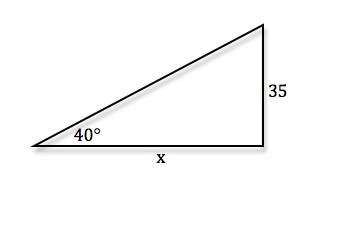• Use SOHCAHTOA to find the length of the shadow
• tan40° = [35/x] ⇒ x = [35/(tan40°)]
x ≈ 41.7 feet
A ladder 20 feet long leans against the side of a building. Find the height from the top of the ladder to the ground if the angle of elevation of the ladder is 53°.
• Start by drawing a picture using the information you are given
•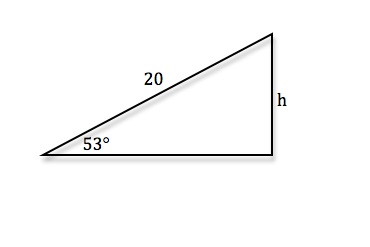• Use SOHCAHTOA to find the height of the ladder to the ground
• sin53° = [h/20] ⇒ h = 20sin53°
h ≈ 15.97 feet
The length of a shadow of a house is 185 feet when the angle of elevation of the sun is 48°. What is the approximate height of the house?
• Start by drawing a picture using the information you are given
•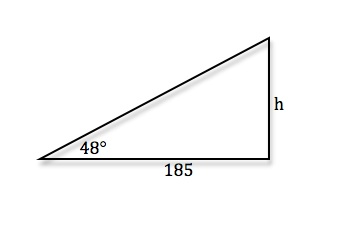• Use SOHCAHTOA to find the height of the house
• tan48° = [h/185] ⇒ h = 185tan48°
h ≈ 205.46 feet
A young girl is flying a kite on 300 feet of string. The kite string makes a 35° angle with the ground. How high is the kite?
• Start by drawing a picture using the information you are given
•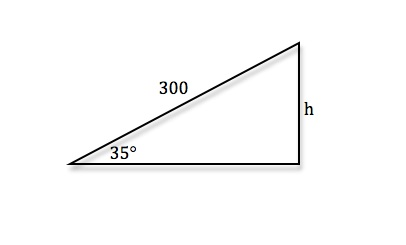• Use SOHCAHTOA to find the height of the kite
• sin35° = [h/300] ⇒ h = 300sin35°
h ≈ 172.1 feet
An engineer erects a 85 - foot vertical tower for an antenna. Find the angle of elevation to the top of the tower at a point on level ground 60 feet from its base.
• Start by drawing a picture using the information you are given
•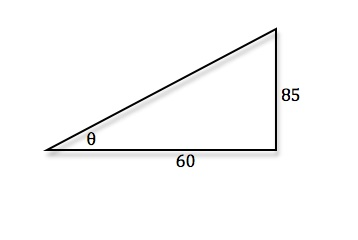• Use SOHCAHTOA to find the angle of elevation
• tanθ = [85/60] ⇒ θ = arctan([85/60])
θ ≈ 54.8°
The sonnar of a navy cruiser detects a submarine that is 3500 feet from the cruiser. The submarine is 2237 feet deep. Find the angle between the water line and the submarine.
• Start by drawing a picture using the information you are given
•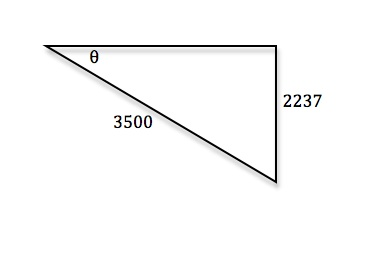• Use SOHCAHTOA to find the length of the shadow
• sinθ = [2237/3500] ⇒ θ = arcsin([2237/3500])
θ ≈ 39.7°
A bridge is built across a lake from A to B. The bearing from B to A is S33°W. From point C, 150 meters from B, the bearings to B and A are S67°E and S18°E respectively. Find the distance from A to B.
• Start by drawing a picture using the information you are given
•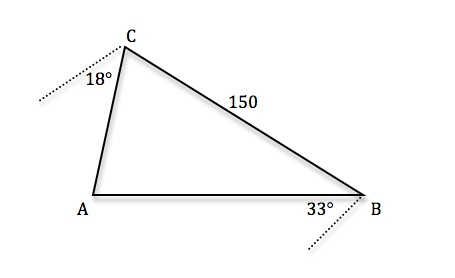• C = 67o − 18o = 49o
• B = 180o − 67o − 33o = 80o
• A = 180o − 49o − 80o = 51o
•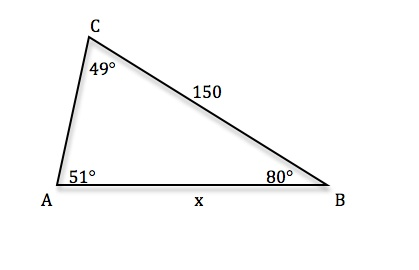• Use Law of Sines
• [(sin51°)/150] = [(sin49°)/c] ⇒ c  sin51° = 150sin49° ⇒ c = [(150sin49°)/(sin51°)]
c ≈ 146 meters
Due to very strong winds, a Magnolia Tree grew so that it is leaning 8° from the vertical. At a point 45 meters from the tree, the angle of elevation is 32°. Find the height of the tree.
• Start by drawing a picture using the information you are given
•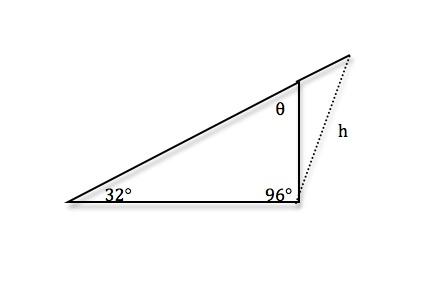• θ = 180° − 32° − 96° = 52°
• Use Law of Sines
• [(sin32°)/h] = [(sin52°)/45] ⇒ h sin52° = 45sin32° ⇒ c = [(45sin32°)/(sin52°)]
h ≈ 30.3 meters
A triangular piece of land has sides of length 730 feet, 620 feet, and 560 feet. Find the measure of the largest side.
• Start by drawing a picture using the information you are given
•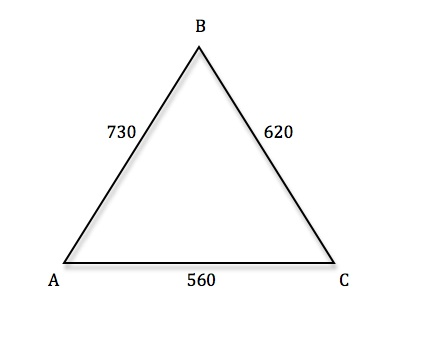• Use the Law of Cosines
• cosC = [(a2 + b2 − c2)/2ab] ⇒ cosC = [(6202 + 5602 − 7302)/2(620)(560)] ⇒ C = arccos([165100/694400])
C ≈ 76.2°
A land surveyor measures the fences along the edges of a triangular field to be 480 feet, 540 feet, and 570 feet. What is the area of the field?
• Start by drawing a picture using the information you are given
•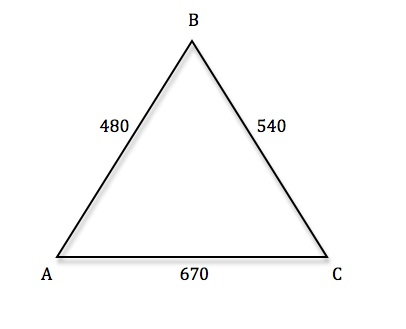• Use Heron's Formula to find the area: A = √{s(s − a)(s − b)(s − c)} ⇒ where s = [1/2](a + b + c)
• s = [1/2](480 + 540 + 670) ⇒ s = 845
• A = √{845(845 − 480)(845 − 540)(845 − 670)} ⇒ A = √{845(365)(305)(175)}
A ≈ 128305.04 square feet

*These practice questions are only helpful when you work on them offline on a piece of paper and then use the solution steps function to check your answer.

### Word Problems and Applications of Trigonometry

Lecture Slides are screen-captured images of important points in the lecture. Students can download and print out these lecture slide images to do practice problems as well as take notes while watching the lecture.

• Intro 0:00
• Formulas to Remember 0:11
• SOHCAHTOA
• Law of Sines
• Law of Cosines
• Heron's Formula
• Example 1: Telephone Pole Height 4:01
• Example 2: Bridge Length 7:48
• Example 3: Area of Triangular Field 14:20
• Extra Example 1: Kite Height
• Extra Example 2: Roads to a Town

### Transcription: Word Problems and Applications of Trigonometry

We are trying some more examples of applied trigonometry to solve word problems.0000

In this first one, we are given a child flying a kite on 200ft of string and the kite’s string makes an angle of 40 degrees with the ground.0006

We want to figure out how high is the kite of the ground.0014

Remember, whenever you have these word problems, the important thing is to draw a picture right away.0019

That helps you convert from lots of words into some triangles or geometric picture where you can invoke the equation that you know.0023

Let me draw this now, the child is flying a kite on 200ft of string and the kite’s string makes an angle of 40 degrees with the ground, that is a 40 degree angle.0034

I want to figure out how high the kite is off the ground so let me draw in something to mark the height here.0050

There is the kite up there and here is the child, we are not going to worry about how tall the child is because that really will not make a difference.0066

We are trying to find this distance right here, that is the height of the kite off the ground.0077

This is a right triangle so we can use our formulas for right triangles which is SOHCAHTOA.0086

Remember SOHCAHTOA only works for right triangles, if you do not have a right triangle then you will going use something like the law of sin or law of cos, or heron’s formula.0092

If you have a right triangle, SOHCAHTOA is usually faster.0102

Let us see what I know here, I do not really know anything except the hypotenuse of this triangle and one angle, and then this is the opposite side to the angle that I know.0108

I’m going to use this part of SOHCAHTOA, sin=opposite/hypotenuse, sin(theta)=opposite/hypotenuse that is because I know the hypotenuse and I’m looking for the opposite side there.0122

Sin(40)=opposite/200 and if I solve that by for the opposite side, I will get the opposite side as equal to (200) sin (40).0140

Let me check on my calculator what that is, since that is not a common value, that is not one that I just remember.0162

What I get is an approximate value of 128.6 and we are told that the unit of measurement here is feet, so, 128.6ft.0171

That one was a pretty quick one, the reason it was quick because we really had a right angle there so we are able to use SOHCAHTOA.0199

If we did not have a right angle and we had to use the law of cos or law of sin, it probably would have been longer.0205

Let us recap what it took to do that problem.0212

First of all, you read the word and try to make a sense of it then you draw a picture.0215

It is very important that you draw a picture to illustrate what is going on.0219

We drew a picture with a child here, the kite here, and we know that the child is flying a kite on 200ft of string.0223

We fill that in, we are told that the kite’s string makes a 40 degree angle with the ground and we draw that in.0233

We are trying to find how high is the kite? We draw in the height of the kite.0240

We have a right angle, definitely a candidate for SOHCAHTOA and the reason I picked sin is because I want to find the opposite side to the angle and I know the hypotenuse.0247

That is why I worked with sin, sin=opposite/hypotenuse.0258

I filled in the values that I know 40 and 200, and I just solved it for the opposite.0263

I got a number and then I checked back to see that we are using feet.0268

That tells me that my unit for measurement for the answer should be feet.0274

Our last example here is another pretty wordy one, two straight roads lead from different points along the coast to an in land town.0000

Surveyors working on the coast measure that the roads are 12 miles apart and make angles of 40 degrees and 110 degrees with the coast.0009

I want to find out how far is it to the town along each one of the roads?0019

This is a pretty complicated one, when you get like one like this with lots of words, it is absolutely essential that the first thing you do is draw a picture.0025

Let me draw a picture, that is supposed to be the coastline there.0034

Those are supposed to be fish swimming in the ocean and we have got two different points along the coast.0047

Two roads leads from these points to an in land town and these roads make angles of 40 degrees and 110 degrees with the coast.0055

That is about 110 and that is about 40, we know that straight roads go off and they go to some town somewhere in land.0069

The last thing we are told is that the roads are 12 miles apart, I’m going to write in my third side as 12 miles there.0086

We want to figure out how far it is to the town along in each of the roads.0099

First of all, let us look at this triangle we got here.0104

What I have been given is two angles of the triangle and the side in between them, so we have been given an (angle, side, angle) situation.0108

When you are given an (angle, side, angle) situation, the thing you want to check is whether those angles are really legitimate.0120

In other words, whether they add up to less than 180 degrees.0127

The angles sum up to, in this case (110+40=150) which is less than 180, which is good, there is a unique solution.0132

I’m going to label everything I know here, I’m going to label that unknown angle as (C) and the others are (A and B).0160

I will label my sides (a, b – remember you put the sides opposite the angles of the same letter and that side that we know is 12 is (c).0172

Now I have labeled everything I know and I want to find the length of the roads, that is (a and b), so how can I find those?0189

This is an (angle, side, angle) situation, (angle, side, angle) if you remember that is the one you want to solve using the law of sin.0202

That one you want to solve with the law of sin, it does not work very well with the law of cos.0218

Certainly, it does not work with SOHCAHTOA because we do not have a right angle here.0223

We are going to use the law of sin, let me remind you what that is, that says sin(A)/a=sin(B)/b=sin(C)/c.0227

That is the law of sin and we want to use that to solve for (a) and (b).0245

Let me work on (a), I will write down sin(A)/(a)=sin(C)/c, the reason I’m going to that is because I know what (c) is and I do not know what any of the others are.0251

I can find (C) very easily, (C) is 180-(A)-(B), that is because the three angles of a triangle add up to 180.0271

That is 180-110-40, 180-150=30, so (C) is 30 degrees, that is not my full answer to the problem but that is going to be useful to me.0284

Sin(A)=sin(110), sin(a)= I do not know yet, sin(C)=sin(30), sin(c)=12.0307

I’m going to cross multiply to solve for (a), 12 sin(110)=(a)sin(30), if I solve that for (a), (a)=(12)sin (110)/sin(30).0325

I’m going to reduce that using my calculator, remember to set your calculators on degree mode if that is what you are using.0349

I’m going to simplify (12)sin(110)/sin(30), it tells me that it is approximately 22.6.0364

The unit of measurement here is miles be we were told here that the measurement on the coast was given as 12 miles.0388

That tells us how long the A road is, 22.6 miles.0398

Now let us figure out how long the B road is, again we are using the law of sin.0405

Sin(B)/(b)=sin(C)/sin(c), I fill in what I know there.0409

I know that (B) is 40 degrees, I do not know (b), that is what I’m solving for, sin(C) is sin(30), (c) is (12).0421

I will cross multiply there, I get (12)sin(40)=(B)sin(30), I want to solve for (b), (b)=(12)sin(40)/sin(30).0441

I will plug that into my calculator.0470

Make sure that it is in degree mode and I get an approximate answer of 15.4 miles for (b).0477

Now I have the lengths of those two roads to the town in land.0493

Let us recap what we did there, we were graded with this long problem and lots of words.0498

The first thing to do is draw a picture and try to identify everything you are being given in the problem.0502

We have different points along the coast, we have an in land town, we measure the roads are 12 miles apart.0509

I filled that 12 miles into my distance along the coast, I filled in the two angles, and that was really all the problem gave me.0517

But that was enough to set up a triangle and to notice that I have (angle, side, angle).0529

Once I know that I have (angle, side, angle) I can the measure of the angles, make sure it is less than 180 and that tells me that it has a unique solution.0535

Now with (angle, side, angle) it is really not a good one to work with cos, certainly it does not work with SOHCAHTOA because we do not have a right angle.0545

Remember that SOHCAHTOA only works with right angles.0554

We are stuck using the law of sin, which actually works very well for (angle, side, angle).0558

First thing we had to do is find that third angle, the way we did that was by noticing that the angles add up to 180 degrees.0564

We used that to find the value of the third angle, that the value of angle C is 30 degrees.0572

We were able to plug that in to these incarnations of the law of sines, sin(A)/(a)=sin(C)/(c).0578

We plug in everything we know and the only thing missing is this (a).0588

We were able to solve this down and get (a) equal to 22.6 and we figured out that the unit of measurement is miles.0594

That is why we said that the answer was in term of miles.0603

We used the law of sines again, sin(B)/(b)=sin(C)/(c) and fill in everything we know, reduced it down, solve for (b) and give our answer in terms of miles.0607

That tells us the length of those two roads that finishes off this problem.0620

That also finishes the lecture on word problems and applications of triangle trigonometry.0624

Thanks for watching this trigonometry lectures on www.educator.com.0631

Hi, these are the trigonometry lectures for educator.com, and today we're going to look at some word problems and some applications of triangle trigonometry.0000

We're going to be using all the major formulas that we've learned in the previous lectures.0009

I hope you remember those very well.0014

The master formula which works for right triangles is SOH CAH TOA.0016

You can remember that as Some Old Horse Caught Another Horse Taking Oats Away.0021

Remember, that only works in a right triangle.0025

If you have an angle θ, that relates the sine, cosine and tangent of θ to the hypotenuse, the side opposite θ, and the side adjacent to θ.0030

You interpret that as the sin(θ) is equal to opposite over hypotenuse, the cos(θ) is equal to adjacent over hypotenuse, and the tan(θ) is equal to opposite over adjacent.0043

The law of sines works in any triangle.0056

Let me draw a generic triangle here, doesn't have to be a right triangle for the law of sines to work, so a, b, and c ...0060

Generally, you'd label the angles with capital letters, and label the sides with lowercase letters opposite the corner with the same letter.0071

That makes this a, this is b, and this is c.0082

The law of sines says that sin(A)/a=sin(B)/b=sin(C)/c.0086

That's the law of sines.0106

Law of cosines also works in any triangle.0108

Let me remind you what that one is.0112

We had a whole lecture on it earlier, but just to remind you quickly, it says that c2=a2+b2-2abcos(C).0114

That's useful when you know all three sides, you can figure out an angle very quickly using the law of cosines.0129

Or if you know two sides and the angle in between them, you can figure out that third side using the law of cosines.0134

Remember, law of cosines works in any triangle, doesn't have to be a right triangle.0142

It still works in right triangles.0147

Of course, in right triangles, if C is the right angle, then cos(C) is 0, so the law of cosines just boils down to the Pythagorean formula.0149

You can think of the law of cosines as kind of a generalization of the Pythagorean theorem to any triangle, doesn't have to be a right triangle anymore.0158

Finally, Heron's formula.0166

Heron's formula tells you the area of a triangle when you know the lengths of the three sides.0173

Heron says that the area is equal to the square root of s×(s-a)×(s-b)×(s-c).0178

The a, b, and c are the lengths of the sides, you're supposed to know what those are before you go into Heron's formula.0193

This s I need to explain is the semi-perimeter.0199

You add a, b, and c, you get the perimeter of the triangle, then you divide by 2 to get the semi-perimeter.0205

That tells you what the s is, then you can drop that into Heron's formula and find the area of the triangle.0212

We'll be using all of those, and sometimes it's a little tricky to interpret the words of a problem and figure out which formula you use.0218

The real crucial step there is as soon as you get the problem, you want to draw a picture of the triangle involved, and then see which formula works.0225

Let's try that out on a few examples and you'll get the hang of it.0237

The first example here is a telephone pole that casts a shadow 20 feet long.0241

We're told the sun's rays make a 60-degree angle with the ground.0247

We're asked how tall is the pole.0250

Let me draw that.0252

Here's the telephone pole, and we know it casts a shadow, and we know that that shadow is 20 feet long.0254

That's a right angle.0262

The reasons it casts a shadow is because of these rays coming from the sun.0265

There's the sun casting the shadow.0274

We want to figure out how tall is the pole.0278

We're told that the sun's rays make a 60-degree angle with the ground.0281

That means that angle right there is 60 degrees.0284

We want to solve for the height of the telephone pole, that's the quantity we want to solve for.0288

That's the side opposite the angle that we know.0295

We also are given the side adjacent to the angle we know.0303

I see opposite and adjacent, and I see a right angle.0305

I'm going to use SOH CAH TOA here.0309

I know the adjacent side, I'm looking for the opposite side.0315

It seems like I should use the tangent formula here.0325

Tan(60) is equal to opposite over adjacent.0330

Tan(60), 60 is one of those common values, that's π/3.0339

I know what the tan(60) is, I've got that memorized and hopefully you do too, square root of 3 is the tan(60).0346

If you didn't remember that, I at least hoped that you remember the sin(60) and the cos(60), that tan=sin/cos.0353

You can always work out the tangent if you don't remember exactly what the tan(60) is.0364

The sin(60) is root 3 over 2, the cos(60) is 1/2, that simplifies down to square root of 3.0368

That's what the tan(60) is.0377

The opposite, I don't know what that is, I'll just leave that as opposite, but the adjacent side I was given is 20.0380

I'll solve this opposite is equal to 20 square root of 3.0387

Since this is an applied problem, I'll convert that into a decimal, 20 square root of 3 is approximately 34.6.0394

The unit of measurement here is feet, I'll give my answers in terms of feet.0411

That tells me that the telephone pole is 34.6 feet tall.0420

Let's recap there.0428

We were given a word problem, I don't know at first exactly what it's talking about.0430

First thing I do is I draw a picture, so I drew a picture of my telephone pole, I drew a picture of the shadow then I noticed that's a right triangle, I could use SOH CAH TOA to solve it.0433

I tried to figure out which quantities do I know, which quantities do I not know.0447

I knew the adjacent side, I knew the angle, but I didn't know the opposite side and that seemed like it was going to work well with the tangent formula.0451

I plugged in what I knew, I solved it down using the common value that I knew, and I got the answer.0461

In the next one, we're trying to build a bridge across a lake but we can't figure out how wide the lake is, because we can't just walk across the lake to measure it.0469

It says that these engineers measure from a point on land that is 280 feet from one end of the bridge, 160 feet from the other.0481

The angle between these two lines is 80 degrees.0490

From that, we're supposed to figure out what the bridge will be, or how long the bridge will be.0494

Lots of words here, it's a little confusing when you first encounter this because there's just so many words here and there's no picture at well.0498

Obviously, the first thing we need to do is to draw a picture.0504

I had no idea what shape this lake is really but I'm just going to draw a picture like that.0513

I know that these engineers are trying to build a bridge across it.0523

Let's say that that's one end of the bridge right there and that's the other end.0529

They measure from a point on land that is 280 feet from one end of the bridge and 160 feet from the other.0532

It says the angle between these two lines measures 80 degrees.0551

If you look at this, what I have is a triangle, and more than that I have two sides and the included angle of a triangle.0557

I have a side angle side situation, and I know that that gives me a unique solution as long as my angle is less than 180 degrees.0566

Of course, 80 is less than 180 degrees.0576

I know I have a unique solution.0580

I'm trying to find the length of that third side.0585

If you have side angle side and you need the third side, that's definitely the law of cosines.0590

I'm going to label that third side little c, and call this capital C, label the other sides a and b.0595

Now, the law of cosines is my friend here, c2=a2+b2-2abcos(C).0605

We know everything there except for little c.0623

I'm just going to plug in the quantities that I know and reduce down and solve for little c.0626

Let me plug in c2, I don't know that yet, a2 is 1602, plus b2 is 2802, minus 2×160×280×cos(C), the angle is 80 degrees.0634

I don't know exactly what that is but I can find that on my calculator.0658

1602=25600, 2802=78400, 2×160×280, I worked that out as 89600, the cos(80), I'll do that on my calculator ...0664

Remember to put your calculator in degree mode if that's what you're using here.0692

A lot of people have their calculator set in radian mode, and then that gives you strange answers, because your calculator would be interpreting that as 80 radians.0694

It's very important to set your calculator to 80 degrees.0704

Cos(80)=0.174, let's see, 25600+78400=104000, 89600×0.174=15559, that's approximate of course, if we simplify that, we get 88441.0710

That's c2, I'll take the square root of that, c is approximately equal to 297.4.0761

Our unit of measurement here is feet, so I'll give my answer in terms of feet.0778

Let's recap what made that one work.0791

We're given this kind of long paragraph full of words and it's a little hard to discern what we're supposed to be doing.0794

First thing we see is, okay, it's a lake, so I drew just a random lake, it's a bridge across a lake, so I drew a picture.0800

That's really the key ideas to draw a picture.0808

I drew my bridge across the lake here.0810

That's the bridge right there.0813

It says we measure from a point that is 280 feet from one end of the bridge, and 160 feet from the other.0817

I drew that point and I filled in the 160 and the 280.0825

Then it gave me the angle between those two lines, so I filled that in.0831

All of a sudden, I've got a standard triangle problem, and moreover, I've got a triangle problem where I know two sides and the angle between them, and what I want to find is the third side of the triangle.0835

That's definitely a law of cosines problem.0847

I write down my law of cosines, I filled in all the quantities that I know then I simplified down and I solved for the answer on that.0851

We'll try another example here.0862

This time, a farmer measures the fences along the edges of a triangular field as 160, 240 and 380 feet.0864

The farmer wants to know what the area of the field is.0873

Just like all the others, I'll start out right away by drawing a picture.0876

It's a triangular field, my picture's probably not scaled, that doesn't really matter, 160, 240 and 380.0885

I have a triangle and I want to figure out what the area is.0895

If you have three sides of a triangle and you want to find the area that's pretty much a dead give-away that you want to use Heron's formula.0900

Let me remind you what Heron's formula is.0907

Heron's formula says the area is equal to the square root of s×(s-a)×(s-b)×(s-c).0910

The a, b, and c here just the lengths of the three sides but this s is the semi-perimeter of the triangle.0929

That's 1/2 of the perimeter a+b+c.0939

The perimeter is the distance around if you kind of walk all the way around this triangle.0945

That's (1/2)(160+240+300), 160+240=400, plus 300 is 700, 1/2 of that is 350, so s is 350.0949

I just drop the s and the three sides into Heron's formula.0968

That's 350×(350-160)×(350-240)×(350-300), (350-160)=190, (350-240)=110, and (350-300)=50.0976

I can pull 100 out of that immediately, so this is 100 times the square root of 35×19×11×5.1014

I'm going to go to my calculator for that, 35×19×11×5=36575, square root of that is 191, times 100 is 19.1, rounds to 25.1026

This is the area of a field, the units are squared here, and we were talking in terms of feet, so this must be square feet.1060

Let's recap how you approach that problem.1081

First of all, you read the words and right away you go to draw a picture, I see that I have a triangular field, the edges are 160, 240 and 300 feet.1086

I'm asked for the area of the field.1099

Now, once you have the three sides and you want the area, there's pretty much no question that you want to use Heron's formula on that.1101

That's the formula that tells you the area based on the three sides very quickly.1109

You write down Heron's formula, that's got a, b and c there.1115

It's also got this s, the s is the semi-perimeter.1118

You figure that out from the three sides.1122

You plug that into Heron's formula and you plug in the three sides.1126

The a, b and c are 160, 240 and 300.1133

Then, it's just a matter of simplifying down the numbers until you get an answer and figuring out that the units have to be square feet, because the original measurements in the problem were in terms of feet, and we're describing an area now, it must be square feet.1137

We'll try some more examples later.1153

OR

### Start Learning Now

Our free lessons will get you started (Adobe Flash® required).